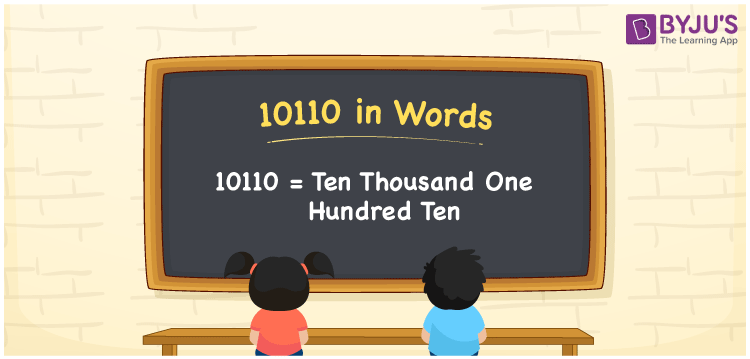# 10110 in Words

10110 in words is written as Ten thousand one hundred ten. In both the International System of Numerals and the Indian System of Numerals, 10110 is written as Ten thousand one hundred ten. The number 10110 is a Cardinal Number as it represents some quantity. For example, “that mobile phone costs 10110 rupees”.

 10110 in Words Ten thousand one hundred ten Ten thousand one hundred ten in Number 10110

## 10110 in English Words

10110 in English words is read as “Ten thousand one hundred ten”.## How to Write 10110 in Words?

To write 10110 in words, we shall use the place value chart. In the place value chart, put 1 in the ten thousand, 0 in the thousands, 1 in the hundreds, 1 in the tens and 0 in the ones. Let us make a place value chart to write the number 10110 in words.

 Ten Thousands Thousands Hundreds Tens Ones 1 0 1 1 0

Thus, we can write the expanded form as

1 × Ten Thousand + 0 × Thousand + 1 × Hundred + 1 × Ten + 0 × One

= 1 × 10000 + 0 × 1000 + 1 × 100 + 1 × 10 + 0 × 1

= 10000 + 0 + 100 + 10 + 0

= 10110

= Ten thousand one hundred ten.

10110 is a natural number, the successor of 10109 and the predecessor of 10111.

10110 in words – Ten thousand one hundred ten

• Is 10110 an odd number? – No
• Is 10110 an even number? – Yes
• Is 10110 a perfect square number? – No
• Is 10110 a perfect cube number? – No
• Is 10110 a prime number? – No
• Is 10110 a composite number? – Yes

## Frequently Asked Questions on 10110 in Words

Q1

### How to write 10110 in words?

10110 in words is written as Ten thousand one hundred ten
Q2

### How to write 10110 in the International and Indian System of Numerals?

In both, the system of numerals, 10110 in words, is written as Ten thousand one hundred ten.
Q3

### How to write 10110 in a place value chart?

In the place value chart, write 1 in the ten thousand, 0 in the thousands, 1 in the hundreds, 1 in the tens and 0 in the ones.